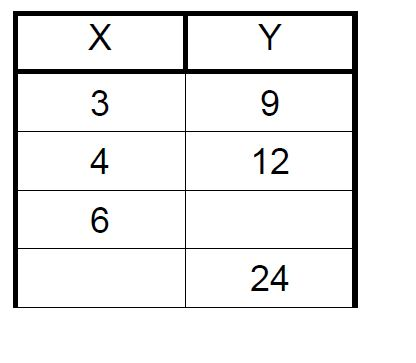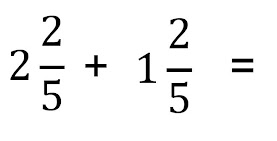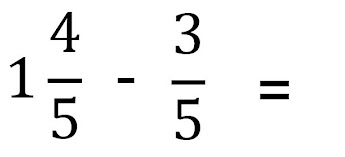4.Q2.W8 Math Quiz: FRIDAY
Name (First and Last) *
1. Compare the numbers using >, <, or = 83,279 ______ 83,322 *
1 point
2. Compare the numbers using >, <, or = 728,485 ______ 782,485 *
1 point
3. Compare the numbers using >, <, or = 1,305,685 ______ 1,053,685 *
1 point
4. 2,548 x 7 = *
1 point
5. Which two numbers would complete the table below? *
1 point6. Find the rule for the table. *
1 point7. Which of the following fractions is equivalent to 5/6? *
1 point
8. Which of the following fractions is equivalent to 10/12? *
1 point
9. Rewrite the improper fraction 4/3 as a mixed number. *
1 point
10. Rewrite the improper fraction 9/4 as a mixed number. *
1 point
11. Find the sum. *
1 point12. Find the difference. *
1 point